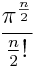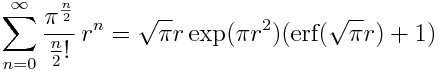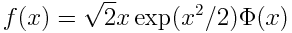# An incomplete post about sphere volumes

This is an incomplete blog post. Maybe you can help finish it.

One of the formulas I’ve looked up the most is the volume of a ball in n dimensions. I needed it often enough to be aware of it, but not often enough to remember it. Here’s the formula:The factor of rn is no surprise: of course the volume as a function of radius has to be proportional to rn. So we can make the formula a little simpler by just remembering the formula for the volume of a unit ball.

Next, we can make the formula a simpler still by using factorials instead of the gamma function. If n is a non-negative integer, n! = Γ(n+1). We can use that to define factorial for non-integers. Then the volume of a unit ball isThat’s easier to remember.

It’s also curious. The nth term in the series for ex is xn/n!, so the volumes of unit balls look like series for eπ except compressed, with each index n cut in half. The volumes are not the coefficients in the series for ex, but could they be the coefficients in the series for another familiar function? To find out, let’s stick back in the factor of rn and sum.This is the sum of the volumes of balls of radius r in all dimensions. That doesn’t make sense by itself, but you could also think of this as the generating function for the volumes of unit balls. So can we find a closed-form expression for the generating function? Yes:If you work with probability, you probably find Φ more familiar than the error function (see notes relating these) and find exp(x2/2) more familiar than exp(x2). So you could rewrite the generating function as f(√(2π)r) whereThat looks familiar, but I don’t know what to do with it.

I warned you this would an incomplete post. I feel like there’s an interesting connection to be made, but I’m not quite there. Any suggestions?

Update: I completely forgot about this post and wrote a more complete post on the same topic on May 26, 2019. As Greg Egan points out in the comments below, there’s an error in this post that the newer post corrects.

## 13 thoughts on “An incomplete post about sphere volumes”

1. Niclas Wiberg

Minor observation:
exp(pi) – 1 = volume sum of all unit balls in even dimensions

2. The formula I can always remember is $int_{-infty}^infty e^{-pi x^2} dx = 1$, from which by Jacobian determinant and symmetry $int_mathbb{S^n} int_0^infty r^n e^{-pi r^2} dr dOmega = 1$. I don’t know if that helps you much, but it does the job.

3. Shae Erisson

Is Coxeter’s Introduction to Geometry the best resource for learning more about n-dimensional solids like this?

4. $$f(x) = phi(x)/(frac{dphi(x)}{dx}/(sqrt{2}x))$$

the value of something as a fraction of the ratio of its derivative to a linear function…

5. Jan Van lent

Michael Hartl posted some comments on the formula for the volume of an n-sphere in a discussion about his Tau Manifesto . He also mentions this at the end of the the talk [2,3].
If you know the values for the 1-sphere (length of interval [-1, 1]) and the 2-sphere (area of unit circle) then the following recursion is easy to remember:
V = 1, V = pi, V[n] = V[n-2] * (2*pi)/n
There is also a very nice recursion that links the volume V[n] and the surface area S[n] .

6. Pseudonym

I think the reason why it looks familiar is that it’s almost the derivative of e^(-x^2/2) Phi[x]. In fact, I believe that f(x) = A d/dx [ e^(-x^2/2) Phi[x] ] + B for some constants A and B.

7. GlennF

I’ve seen this before… I had to reach back to remember this one. Eventually I found it:
American Mathematical Monthly, Vol. 107, No. 3, Mar., 2000, pp. 256-258
“Generating the measures of n-Balls”

He calls the generating function b(r) and makes a nice point about b'(r) being the generator for surface areas of n-spheres. Unfortunately this doesn’t really help you complete the post – the article stops at more or less the same point as the incomplete post does, I think.

8. GlennF

One other comment besides the reference… the form of the generating function is suggestive of some connection between n-spheres and the ordinary 1-dimensional normal distribution. I am aware of another connection of this sort: If one seeks a joint distribution of n i.i.d. real random variables that is also isotropic (spherical symmetry) then that distribution must be the multivariate normal formed by the products of identical 1-d normal distributions.
This is suggestive to me of successive constructions of n-spheres and their volumes (or surface areas), but I’m not sure where to go with it to connect close the loop. Hope this is helpful and not a red-herring.

9. Douglas Muirden

Thank you for this thought-provoking post, I enjoy your blog & take a look now & again.
Can I ask how you arrived at your closed-form for the generating function? I think there may have been some typo and in terms of x it should be 2*exp(x^2/2)*Phi(x). Or maybe better sqrt(2/pi)*Phi(x)/phi(x), cf the Mills ratio.
Anyway thanks again for the post which was even better for being open-ended.

10. In the same period you wrote this post, I inserted a similar one in my blog: http://zibalsc.blogspot.fr/
We had quite the same conclusion, but we found closed-form expression for the generating function with a little difference: http://zibalsc.blogspot.fr/2013/02/115-somma-di-ipersfere.html
The blog is in Italian language, sorry, but you may easy translate it with google translator or simply to look at the formula. Let me know your opinion.

11. I get a result that lacks the overall factor of r√π::

exp(π r^2)(1+erf(r√π))

Mathematica and Wolfram Alpha give the same expression: